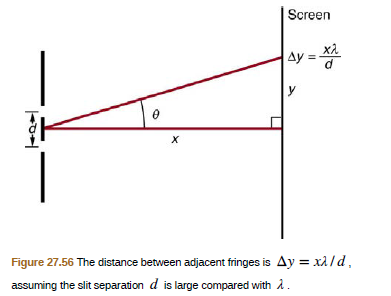Chapter 27, Problem 20PE

Chapter
Section
Textbook Problem

Using the result of the problem two problems prior, find the wavelength of light that produces fringes 7.50 mm apart on a screen 2.00 m from double slits separated by 0.120 mm (see Figure 27.56).To determine

The wavelength of light that produces fringes 7.50 mm apart on a screen 2.00 m from double slits separated by 0.120 mm(see figure 27.56)

Explanation

Given info:

Figure shows a double slit located a distance x, from a screen, with the distance from a screen, with the distance from the center of the screen given by y . When the distance d between the slits is relatively large, there will be numerous bright spots.

Formula used:

Δy=xλdλ=dΔyx

Calculation:

We have, x=2m, Δy=7.50nm, d=0.120 mm

Still sussing out bartleby?

Check out a sample textbook solution.

See a sample solution

The Solution to Your Study Problems

Bartleby provides explanations to thousands of textbook problems written by our experts, many with advanced degrees!

Get Started